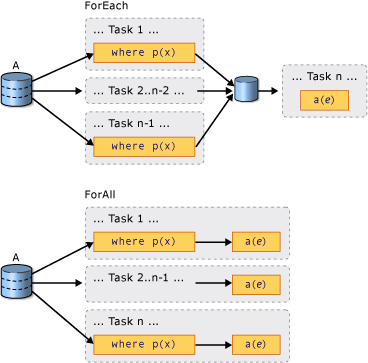# PLINQ 介绍

## ParallelEnumerable 类

System.Linq.ParallelEnumerable 类公开了几乎所有的 PLINQ 功能。 它和 System.Linq 命名空间类型的其余部分一起被编译到 System.Core.dll 程序集中。 Visual Studio 中默认的 C# 和 Visual Basic 项目均会引用该程序集并导入该命名空间。

ParallelEnumerable 运算符 描述
AsParallel PLINQ 的入口点。 指定如果可能，应并行化查询的其余部分。
AsSequential 指定查询的其余部分应像非并行的 LINQ 查询一样按顺序运行。
AsOrdered 指定 PLINQ 应为查询的其余部分保留源序列的排序，或直到例如通过使用 orderby（在 Visual Basic 中为 Order By）子句更改排序为止。
AsUnordered 指定保留源序列的排序不需要查询其余部分的 PLINQ。
WithCancellation 指定 PLINQ 应定期监视请求取消时所提供的取消标记的状态以及取消执行。
WithDegreeOfParallelism 指定 PLINQ 应用于并行化查询的处理器的最大数量。
WithMergeOptions 提供有关 PLINQ 应如何（如果可能）将并行结果合并回使用线程上的一个序列的提示。
WithExecutionMode 指定 PLINQ 应如何并行化查询（即使是当默认行为是按顺序运行查询时）。
ForAll 一种多线程枚举方法，与循环访问查询结果不同，它允许在不首先合并回使用者线程的情况下并行处理结果。
Aggregate 重载 对于 PLINQ 唯一的重载，它启用对线程本地分区的中间聚合以及一个用于合并所有分区结果的最终聚合函数。

## 选择使用模型

var source = Enumerable.Range(1, 10000);

// Opt in to PLINQ with AsParallel.
var evenNums = from num in source.AsParallel()
where num % 2 == 0
select num;
Console.WriteLine("{0} even numbers out of {1} total",
evenNums.Count(), source.Count());
// The example displays the following output:
//       5000 even numbers out of 10000 total

Dim source = Enumerable.Range(1, 10000)

' Opt in to PLINQ with AsParallel
Dim evenNums = From num In source.AsParallel()
Where num Mod 2 = 0
Select num
Console.WriteLine("{0} even numbers out of {1} total",
evenNums.Count(), source.Count())
' The example displays the following output:
'       5000 even numbers out of 10000 total


AsParallel 扩展方法将后续查询运算符（在此示例中为 whereselect）绑定到 System.Linq.ParallelEnumerable 实现。

## 并行度

var query = from item in source.AsParallel().WithDegreeOfParallelism(2)
where Compute(item) > 42
select item;

Dim query = From item In source.AsParallel().WithDegreeOfParallelism(2)
Where Compute(item) > 42
Select item


## 已排序和未排序的并行查询

var evenNums =
from num in numbers.AsParallel().AsOrdered()
where num % 2 == 0
select num;

Dim evenNums = From num In numbers.AsParallel().AsOrdered()
Where num Mod 2 = 0
Select num



## ForAll 运算符

var nums = Enumerable.Range(10, 10000);
var query =
from num in nums.AsParallel()
where num % 10 == 0
select num;

// Process the results as each thread completes
// and add them to a System.Collections.Concurrent.ConcurrentBag(Of Int)
// which can safely accept concurrent add operations

Dim nums = Enumerable.Range(10, 10000)
Dim query = From num In nums.AsParallel()
Where num Mod 10 = 0
Select num

' Process the results as each thread completes
' and add them to a System.Collections.Concurrent.ConcurrentBag(Of Int)
' which can safely accept concurrent add operations## 取消

PLINQ 在 .NET 中与取消类型集成在一起。 （有关详细信息，请参阅托管线程中的取消。）因此，与顺序 LINQ to Objects 查询不同，可以取消 PLINQ 查询。 若要创建可取消 PLINQ 查询，请在查询中使用 WithCancellation 运算符，并提供 CancellationToken 实例作为参数。 如果令牌上的 IsCancellationRequested 属性设置为 true，PLINQ 就会注意到它，停止处理所有线程并抛出 OperationCanceledException

## 自定义分区程序

int[] arr = new int;
Partitioner<int> partitioner = new MyArrayPartitioner<int>(arr);
var query = partitioner.AsParallel().Select(SomeFunction);

Dim arr(10000) As Integer
Dim partitioner As Partitioner(Of Integer) = New MyArrayPartitioner(Of Integer)(arr)
Dim query = partitioner.AsParallel().Select(Function(x) SomeFunction(x))


PLINQ 支持固定数量的分区（尽管在运行时期间为了负载均衡可能会将数据重新动态分配到这些分区）。 ForForEach 仅支持动态分区。也就是说，分区数在运行时发生变化。 有关详细信息，请参阅 PLINQ 和 TPL 的自定义分区程序